# SQL中的条件判断函数CASE WHEN,和IF的用法

1、IF( expr , v1 , v2 )

IF()的返回值为数字值或者字符串，具体情况视其所在语境而定。

select if(1>2,2,3),
if(1<2,'yes','no'),
if(strcmp('test','yes'),'no','yes');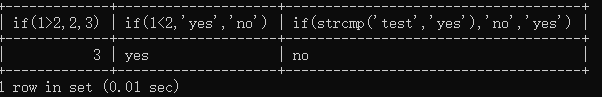select*,if(year(sbirthday)='1976','过关','淘汰') as compete_result from  student where class='95033'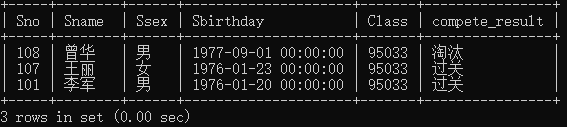2、IFNULL(V1,V2) 函数

select ifnull(1,2),ifnull(null,10),ifnull(1/0,'wrong');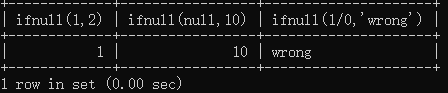3、CASE函数

CASE expr WHEN v1 THEN r1 [WHEN v2 THEN r2] [ELSE rn] END


select sno,cno,degree,
(case when degree>=90 then '优秀'
when degree<90 and degree>70 then '中等'
when degree<=70 and degree>=60 then '及格'
else '不合格' end) as remark
from
score;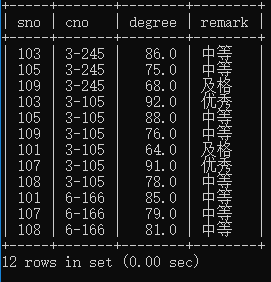THEN后边的值与ELSE后边的值类型应一致，否则会报错。如下：

CASE SCORE WHEN 'A' THEN '优' ELSE 0 END


'优’和0数据类型不一致则报错：

[Err] ORA-00932: 数据类型不一致: 应为 CHAR, 但却获得 NUMBER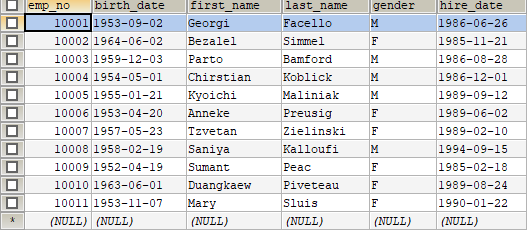select
sum(case when gender='F' then 1 else 0 end) as male_gender,
sum(case when gender='M' then 1 else 0 end) as female_gender,
sum(case when year(birth_date)='1953' and gender='F' then 1 else 0 end) as male_pass,
sum(case when year(birth_date)='1953' and gender='M' then 1 else 0 end) as female_pass
from
employees;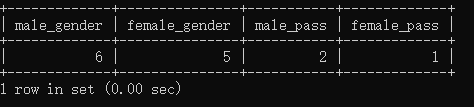例6、经典行转列，并配合聚合函数做统计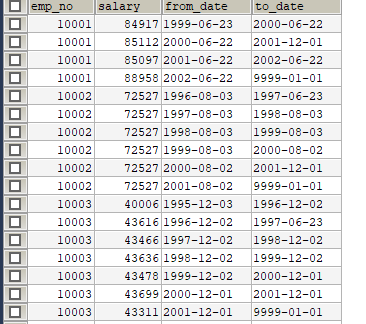select emp_no,
sum(case when to_date='9999-01-01' then salary else 0 end) as a,
sum(case when to_date='2001-12-01' then salary else 0 end) as b,
sum(case when to_date='1997-06-23' then salary else 0 end) as c
from
salaries
group by emp_no;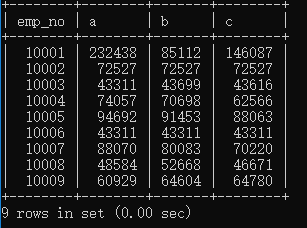02-162万+
05-2514万+10-31158
11-241万+
02-14842
07-264407
12-255898
07-261万+
12-091717
10-204743